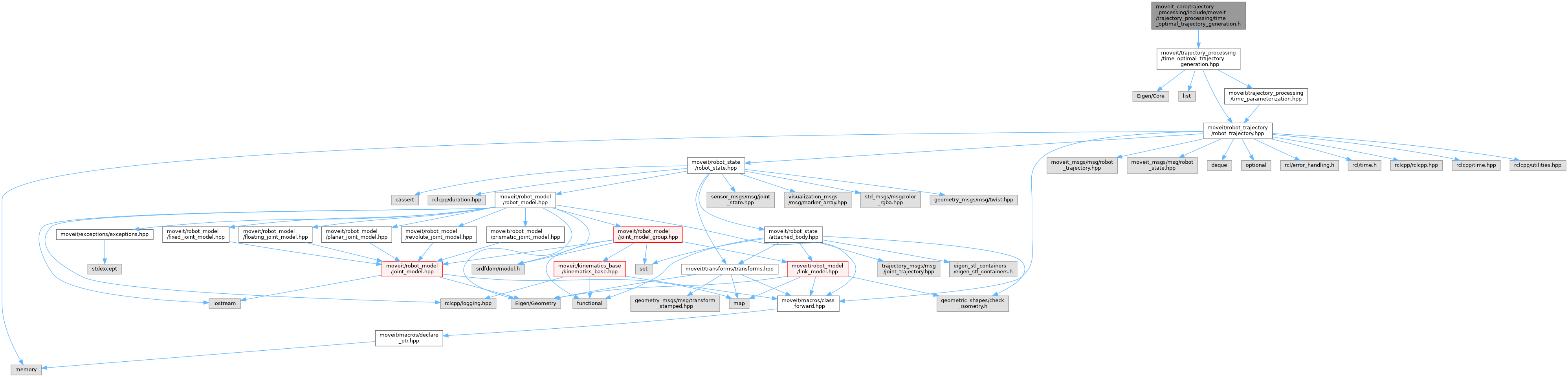moveit2 The MoveIt Motion Planning Framework for ROS 2.
time_optimal_trajectory_generation.h File Reference
`#include <Eigen/Core>`
`#include <list>`
`#include <moveit/robot_trajectory/robot_trajectory.h>`
`#include <moveit/trajectory_processing/time_parameterization.h>`
Include dependency graph for time_optimal_trajectory_generation.h:This graph shows which files directly or indirectly include this file:Go to the source code of this file.

## Classes

class  trajectory_processing::PathSegment

class  trajectory_processing::Path

class  trajectory_processing::Trajectory

class  trajectory_processing::TimeOptimalTrajectoryGeneration

## Namespaces

trajectory_processing

## Enumerations

enum  trajectory_processing::LimitType { trajectory_processing::VELOCITY , trajectory_processing::ACCELERATION }

## Functions

trajectory_processing::MOVEIT_CLASS_FORWARD (TimeOptimalTrajectoryGeneration)

bool trajectory_processing::totgComputeTimeStamps (const size_t num_waypoints, robot_trajectory::RobotTrajectory &trajectory, const double max_velocity_scaling_factor=1.0, const double max_acceleration_scaling_factor=1.0)
Compute a trajectory with the desired number of waypoints. Resampling the trajectory doesn't change the start and goal point, and all re-sampled waypoints will be on the path of the original trajectory (within path_tolerance_). However, controller execution is separate from MoveIt and may deviate from the intended path between waypoints. path_tolerance_ is defined in configuration space, so the unit is rad for revolute joints, meters for prismatic joints. This is a free function because it needs to modify the const resample_dt_ member of TimeOptimalTrajectoryGeneration class. More...

## Variables

const std::unordered_map< LimitType, std::string > trajectory_processing::LIMIT_TYPES